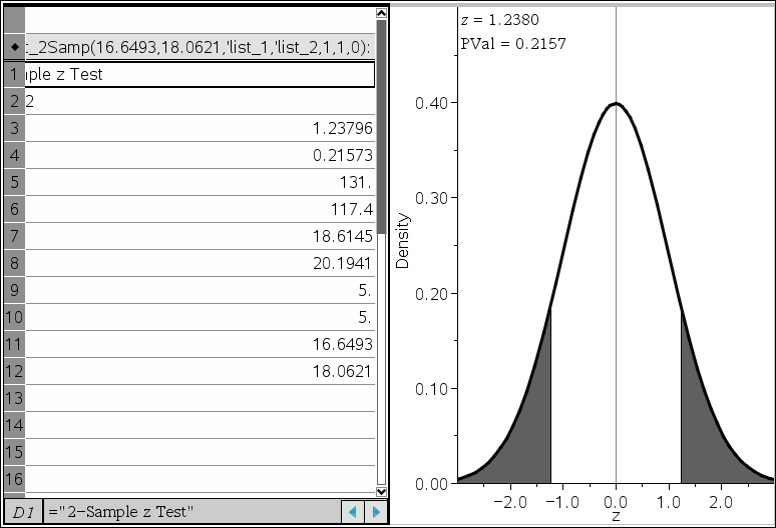# Knowledge Base

## Solution 29610: Performing A 2-Samp Z Test on the TI-Nspire™ Family Products.

### How do I calculate a 2-Samp Z Test using the TI-Nspire family products?

2-Samp Z Tests (two-Sample Z Test) test the equality of the means of two populations (u1 and u2) based on independent samples when both population standard deviations (1 and 2) are known. Using the Lists & Spreadsheets Application, you can calculate all of these values and have the P value shade. Given two lists of data points, the following example demonstrates how to perform a 2-Samp Z Test Calculation using any of the TI-Nspire Handhelds.

Example:

List 1: {154, 109, 137, 115, 140}
List 2: {108, 115, 126, 92, 146}
Population Standard Deviation 1: 16.6493
Population Standard Deviation 2: 18.0621

Solution: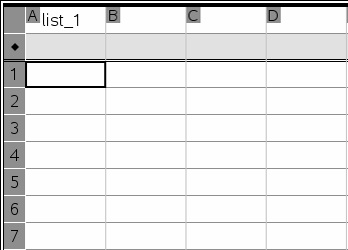2) Scroll to the top of column A, and input "list_1" in the cell with the "A" label. To input an underscore, press [ctrl] + the space bar.
3) Input the numbers from list 1 (given in this example) into the column named "list_1'.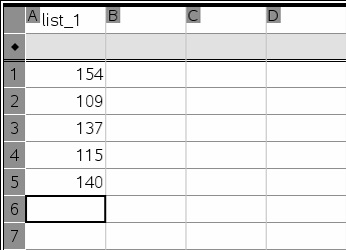4) Scroll to the top of column B, and name it "list_2". In this column, input the values in the example above for list 2.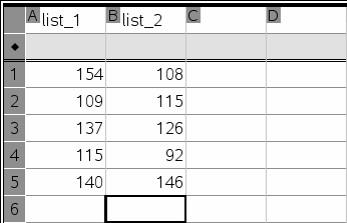5) To select the 2-Samp Z Test, press Menu, then choose "Statistics", choose "Stat Tests" and finally choose "2-Sample Z Test".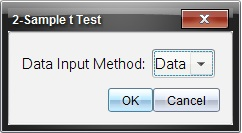6) The Data Input Method should be set to "Data" since we want to perform the test based upon the data we've entered into Lists & Spreadsheet.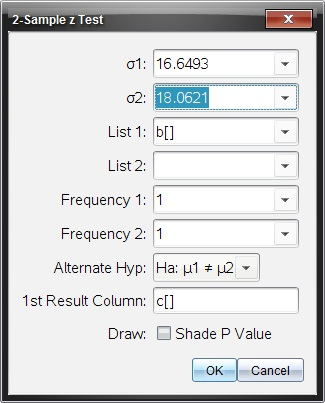7) In the 2-Sample z Test dialog box that appears, the first entry should be the population standard deviation 1 (16.6493), and the second entry should be the population standard deviation 2 (18.0621).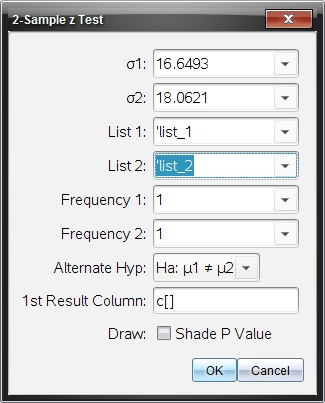8) For list 1, click the appropriate down arrow and choose "'list_1". For list 2, click the appropriate down arrow and choose "'list_2".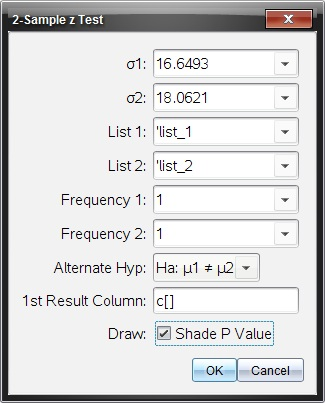9) Both frequencies should be set to 1, the 1st Result Column can be set to c[], and finally click the check box to Shade the P Value. The final result should resemble the following: